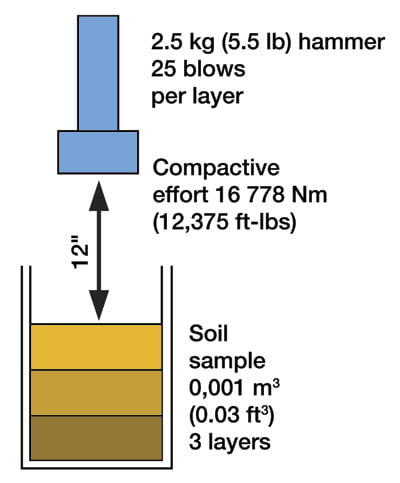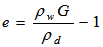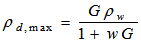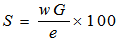# Proctor Test for Soil | Lab Experiment | Advantages & Disadvantages of Proctor Test

### 1. Introduction:

Proctor test for soil is a compaction test that is carried out to determine the compaction properties of soil.

In actuality, this test is used for determining the mass of dry soil per cubic metre when the soil is compacted over a range of moisture contents providing the maximum dry density at the optimum moisture content.

Proctor test includes establishing the relationship between the moisture content and dry density of soils compacted in a mould of a specific size with a 2.5kg rammer dropped from a height of 30cm.

### 2. Apparatus Required:

1. Proctor mould having an internal diameter of 10.2cm and height of 11.6cm with a detachable collar and detachable base plate.

2. A mechanical rammer weighing 2.5kg.

3. Sample extruder

4. Sensitive balance

5. Straight edge

7. Mixing tools such as the spoon, tray, trowel etc.

### 3. Procedures in Proctor Test for Soil:

The procedure for conducting the Proctor compaction test can be listed as follows:

1. Approximately 5kg of the oven-dried sample is taken in the given pan. Water is then poured in it such that the representative sample is dampened four to six percentage points below the optimum moisture content.

2. The Proctor mould is then weighed without the base plate and collar. After that, the collar and base plate are fixed.

3. Place the sample soil in three layers and each layer is compacted by 25 blows from the 2.5kg hammer.

4. The collar is then removed, compacted soil is trimmed with the straight edge and the mould is weighed.

5. The sample is then removed and slightly cut to obtain the sample for moisture content.

6. Then the results are analyzed as follows:

Wet density gm/cc= weight of compacted soil/944

Dry density= wet density/(1+w)

Where,

W= moisture content of the soil

### 4. Observation & Calculation of Proctor Test for Soil

The diameter of the mould =

Height of mould =

The volume of the mould, V=

The specific gravity of solids, G=

 Sl. No. Observations and Calculations Determination No. 1 2 3 Observation 1 Mass of empty mould with base plate 2 Mass of mould compacted soil and base plate Calculations 3 Mass of compacted soil M = (2) – (1) 4 Bulk Density5 Water content, w 6 Dry density7 Void ratio8 Dry density at 100% saturation (theoretical)9 Degree of saturation### 5. Result of Proctor Test

The proctor test determines the capacity of soil with optimal water content to be compacted with a controlled compactive force within that dry unit weight of soil is maximum.

### 6. Precautions in Proctor Test

a. Soil should not contain excess moisture during the compaction process.

b. Dry soil should not be used for this test.

c. Process of compaction should be repeated until there is a decrement of wet unit weight of soil.

d. Clean and fine sieve openings should be used.

e. Mould used for compaction should be cleaned.

### 7. Uses of Proctor Test

a. This test is used to identify the compactness of soil to use in the construction of embankments, highways, foundations, pavements and various earthworks.

b. It is also used to study soil mechanics and other properties like porosity, compressibility, strength, etc.

### 8. Advantages of Proctor Test

a. The compact fill of soil given by the proctor test can aid to determine the degree of soil density.

b. This test also describes the load-bearing capacity of the soil.

c. Due to this test, we can use compacted soils in various engineering works to control soil erosion, reduce subsidence and freeze-thaw problems, etc.

d. It gives the information of water content in the soil with dry unit weight during the compaction procedure.

### 9. Disadvantages of Proctor Test

a. Proctor test fails to determine the density of soil(dry).

b. If soil to be tested contains less moisture, it is difficult to compress the soil.

c. During this test, air voids cannot be removed even after compacting. Thus, the soil is not considered fully saturated.